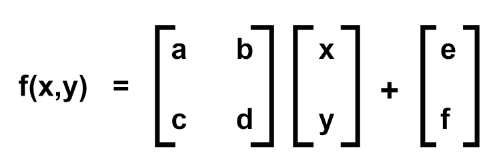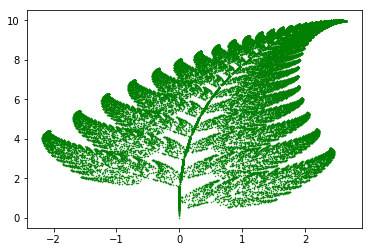# Barnsley Fern in Python

Barnsley fern is a fractal shape created by mathematician Michael Barnsley. The geometric features of this fractal resemble a natural fern and hence it gets its name. Barnsley fern is created by iterating over a large number of times on four mathematical equations, introduced by Barnsley, known as Iterated Function System (IFS).

The transformation Barnsley used had the formula :where, the letters had following value :

 a b c d e f p PART 0 0 0 0.16 0 0 0.01 Stem 0.85 0.04 -0.04 0.85 0 1.60 0.85 Small Leaflet 0.20 -0.26 0.23 0.22 0 1.60 0.07 Large Leaflet(Left) -0.15 0.28 0.26 0.24 0 0.44 0.07 Large Leaflet(Right)

and “p” is the probability.

Thus the four equations are:With the help of above equations, the fern is created. Now lets see the Python3 implementation for the same.

 `# importing necessary modules ` `import` `matplotlib.pyplot as plt ` `from` `random ``import` `randint ` ` `  `# initializing the list ` `x ``=` `[] ` `y ``=` `[] ` ` `  `# setting first element to 0 ` `x.append(``0``) ` `y.append(``0``) ` ` `  `current ``=` `0` ` `  `for` `i ``in` `range``(``1``, ``50000``): ` ` `  `    ``# generates a random integer between 1 and 100 ` `    ``z ``=` `randint(``1``, ``100``) ` ` `  `    ``# the x and y coordinates of the equations ` `    ``# are appended in the lists respectively. ` `     `  `    ``# for the probability 0.01 ` `    ``if` `z ``=``=` `1``: ` `        ``x.append(``0``) ` `        ``y.append(``0.16``*``(y[current])) ` `     `  `    ``# for the probability 0.85     ` `    ``if` `z>``=` `2` `and` `z<``=` `86``: ` `        ``x.append(``0.85``*``(x[current]) ``+` `0.04``*``(y[current])) ` `        ``y.append(``-``0.04``*``(x[current]) ``+` `0.85``*``(y[current])``+``1.6``) ` `     `  `    ``# for the probability 0.07     ` `    ``if` `z>``=` `87` `and` `z<``=` `93``: ` `        ``x.append(``0.2``*``(x[current]) ``-` `0.26``*``(y[current])) ` `        ``y.append(``0.23``*``(x[current]) ``+` `0.22``*``(y[current])``+``1.6``) ` `     `  `    ``# for the probability 0.07     ` `    ``if` `z>``=` `94` `and` `z<``=` `100``: ` `        ``x.append(``-``0.15``*``(x[current]) ``+` `0.28``*``(y[current])) ` `        ``y.append(``0.26``*``(x[current]) ``+` `0.24``*``(y[current])``+``0.44``) ` `         `  `    ``current ``=` `current ``+` `1` `  `  `plt.scatter(x, y, s ``=` `0.2``, edgecolor ``=``'green'``) ` ` `  `plt.show()         `

Output :Note: The whole output depends upon the coefficients of the equations. One experiment might be to change the coefficients and get a new pattern every time.

My Personal Notes arrow_drop_upCheck out this Author's contributed articles.

If you like GeeksforGeeks and would like to contribute, you can also write an article using contribute.geeksforgeeks.org or mail your article to contribute@geeksforgeeks.org. See your article appearing on the GeeksforGeeks main page and help other Geeks.

Please Improve this article if you find anything incorrect by clicking on the "Improve Article" button below.

Article Tags :

2

Please write to us at contribute@geeksforgeeks.org to report any issue with the above content.